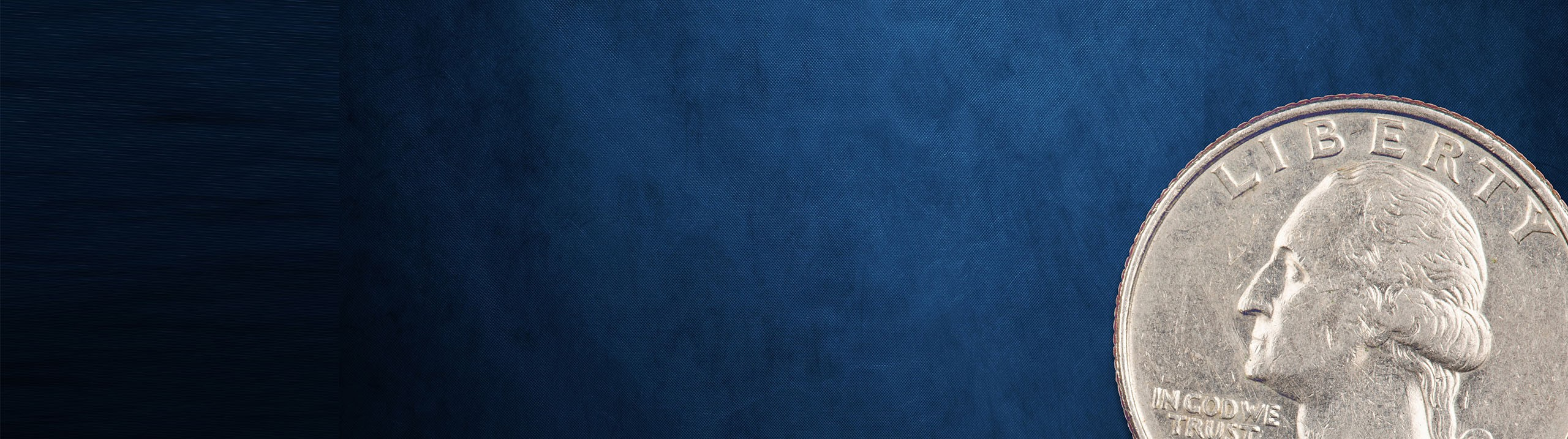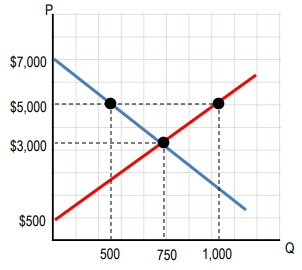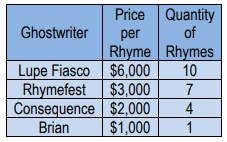Start typing, then use the up and down arrows to select an option from the list.# Microeconomics

Learn the toughest concepts covered in Microeconomics with step-by-step video tutorials and practice problems by world-class tutors

Consumer and Producer Surplus; Price Ceilings and Floors

# Consumer Surplus and Willingness to Pay

## Let's analyze some details about the demand curve.

1

#### concept

Consumer Surplus in a Small Setting14m
Play a video:
2

#### concept

Consumer Surplus and Market Demand6m
Play a video:
3

#### example

Consumer Surplus3m
Play a video:
Alright, let's try this one. The graph below represents the market for funky fresh rhymes at a price of \$3,000 per funky fresh rhymes. What is the consumer surplus? All right, cool. So what do we got here? They're asking us for consumer surplus. Right. We've got a bunch of quantities on the graph, a bunch of prices. We've got our curves there, right? So they're asking us about consumer surplus. And remember that's the area below the demand curve and above the market price. Right? We're gonna get a triangle out of that and it's gonna be this area right here. I'm gonna highlight it in green. So our market price, what was it? \$3,000? And that's right here. So we want to get the area above the market price, but below the demand curve, right, this is our downward demand, right? The double d downward demand right here, let's go ahead and highlight that area. So it's all of this green area right here, right below the demand curve above market price. So how do we calculate that? Well, it is a triangle as you can see, right? So we've got the area of a triangle is gonna be half times the base times the height. Right? So let's go ahead and find out what our base and our height are. So base and height are gonna be these two sides here and this side right here, right? That's gonna we'll call that our base. Either one can be based. Either one can be height, as long as you pick them. All right, so what's gonna be our base, right? Our base is gonna be this length between the 3000 and the 7000. So it's gonna be half times our base is the difference between the 7000 and the 3000. Right? It's gonna be that 4000 difference, but I'm gonna write it out here. 7000 minus 3000, Right? That's that length of of the base. And what's the length of the height here? What do you see here? We've got a bunch of numbers. Well, it's actually gonna be that quantity demanded at that price of 3000. The quantity demanded is 7 50. And that represents this length right? From 0 to 7 50 times 7 50 in that case. Right? There's no subtraction needed there because we're going all the way from zero. So, that's that's pretty much the bulk of it. Let's do this math and figure out what the answer is. So half times 7000 minus 3000 is 4000 times 7 50 right? Uh 4000 times 7 50 three million and half of that is 1.5 million. Right? And that's going to be the area of that triangle, which is our consumer surplus. So, our answer here at a price of \$3000 per funky fresh rhyme. What is consumer surplus? It is going to be be \$1.5 million. Alright, let's go ahead and move on to the next video
4
Problem

Use the graph for funky-fresh rhymes above. If price increases from \$3,000 to \$5,000 per funky-fresh rhyme, what is the change to consumer surplus?5
Problem

Kanye West is ready to create his next hit single. He knows that he is willing to pay up to \$3,000 for a funky fresh rhyme, and that he will need a total of ten funky fresh rhymes to create his hit single. After rounding up his best ghostwriters, he summarized the following schedule. If Kanye values all funky-fresh rhymes equally, what is his maximum consumer surplus?6
Problem

The demand curve for Nickelback’s new album is downward sloping. At a price of \$2, nationwide demand is 100 albums. If the price rises to \$3, what happens to consumer surplus?